# RS Aggarwal Solutions for Class 9 Chapter 1: Number Systems Exercise 1E

## RS Aggarwal Solutions for Class 9 Maths Exercise 1E PDF

Solving exercise wise problems in Mathematics is made easier with the help of RS Aggarwal Solutions for Class 9. The expert faculties having knowledge in Mathematics field help students with solutions in understandable language. Practising exercise wise problems on a daily basis according to RS Aggarwal textbook is important for the students to face the exams without fear. The solutions for class 9 are prepared keeping in mind the marks allotted for each step from exam point of view. Many number of examples and exercise problems are present in order to make it easy for students in solving the problems. RS Aggarwal Solutions for Class 9 Maths Chapter 1 Number Systems Exercise 1E are provided here.

## RS Aggarwal Solutions for Class 9 Chapter 1: Number Systems Exercise 1E Download PDF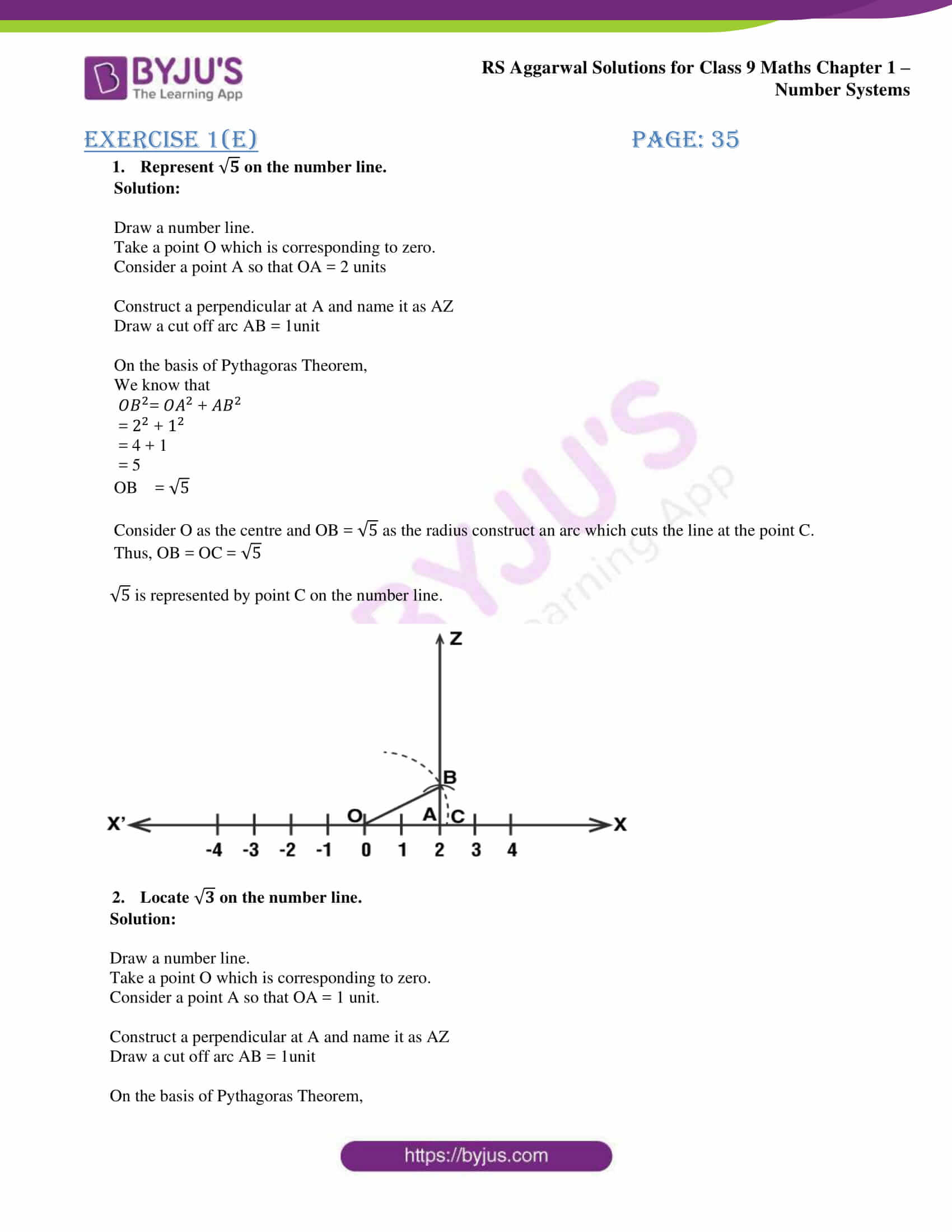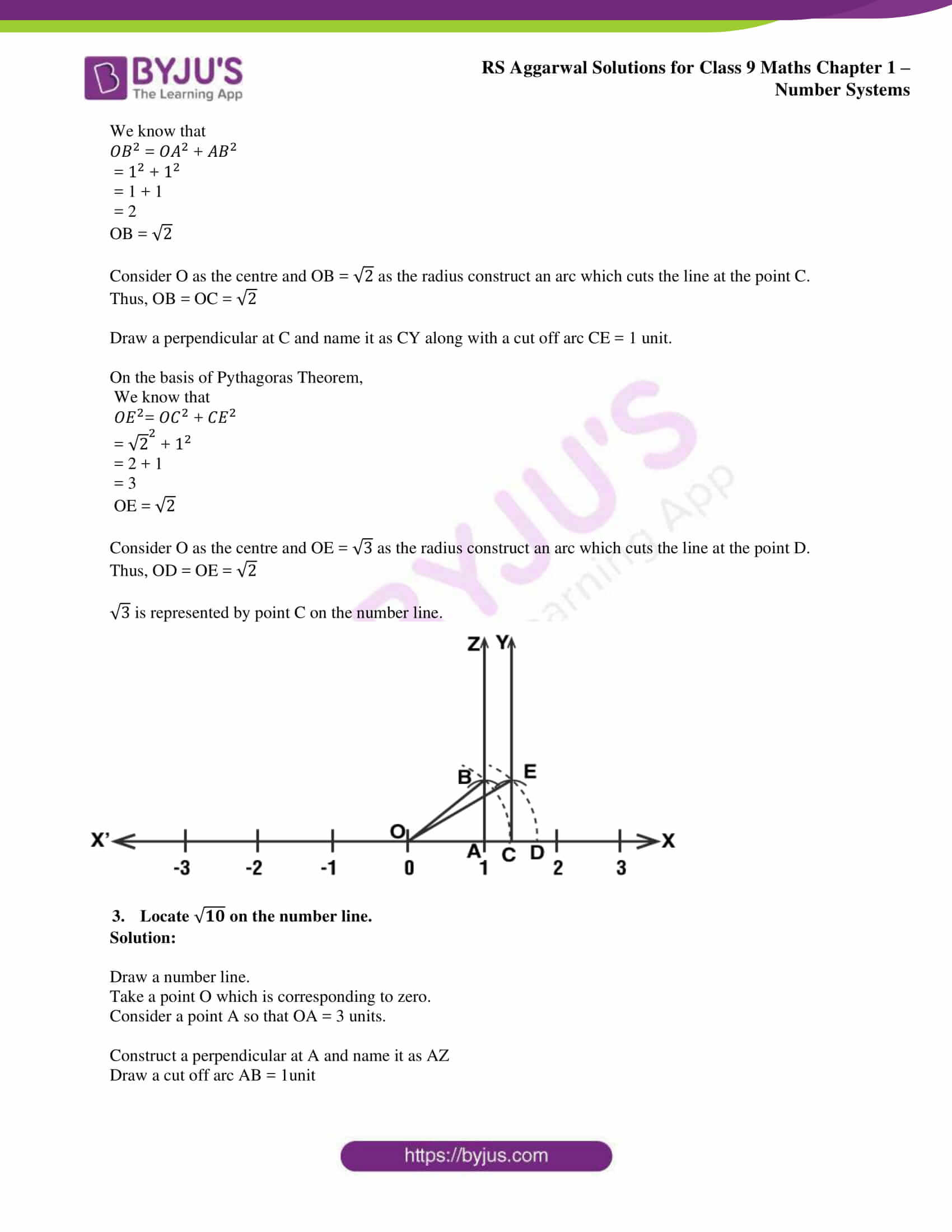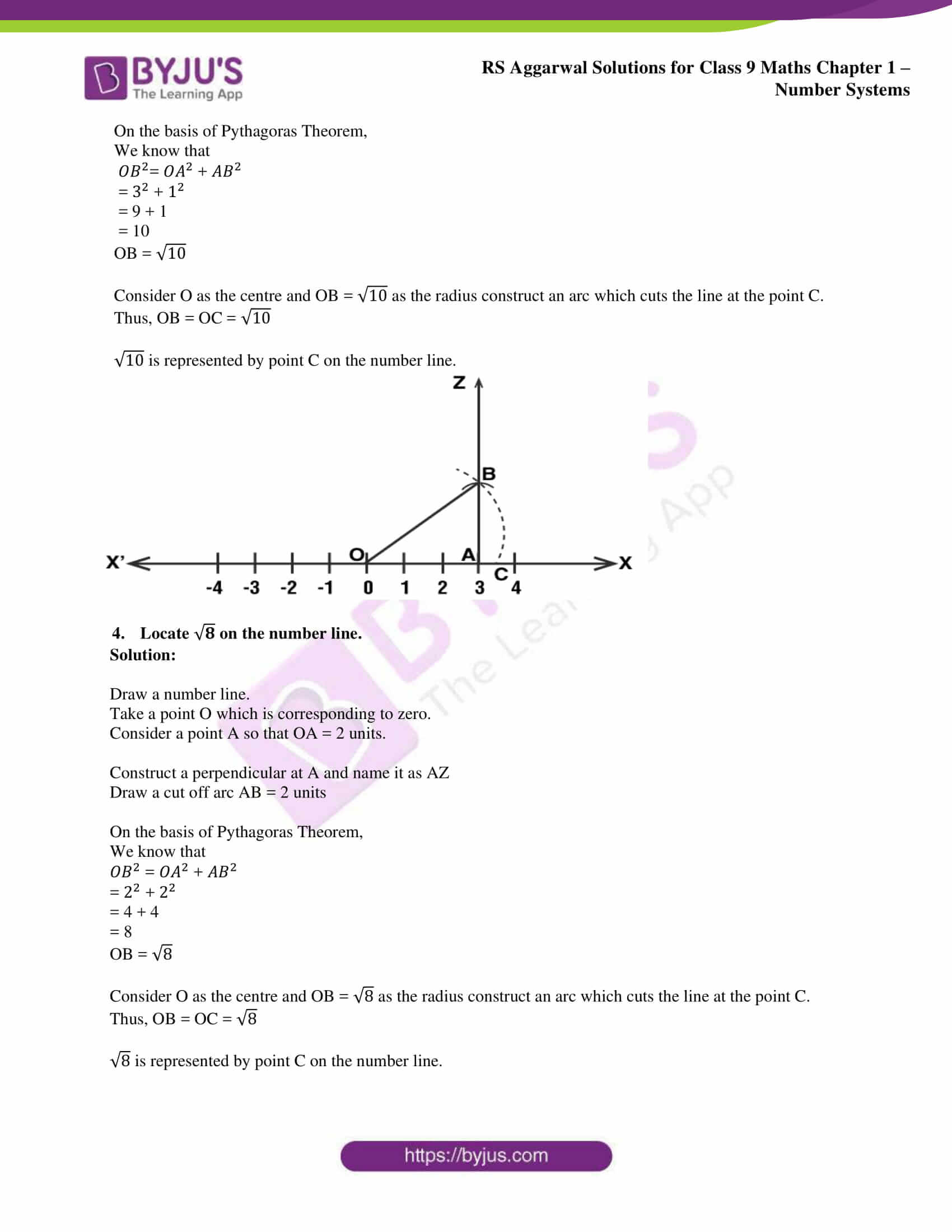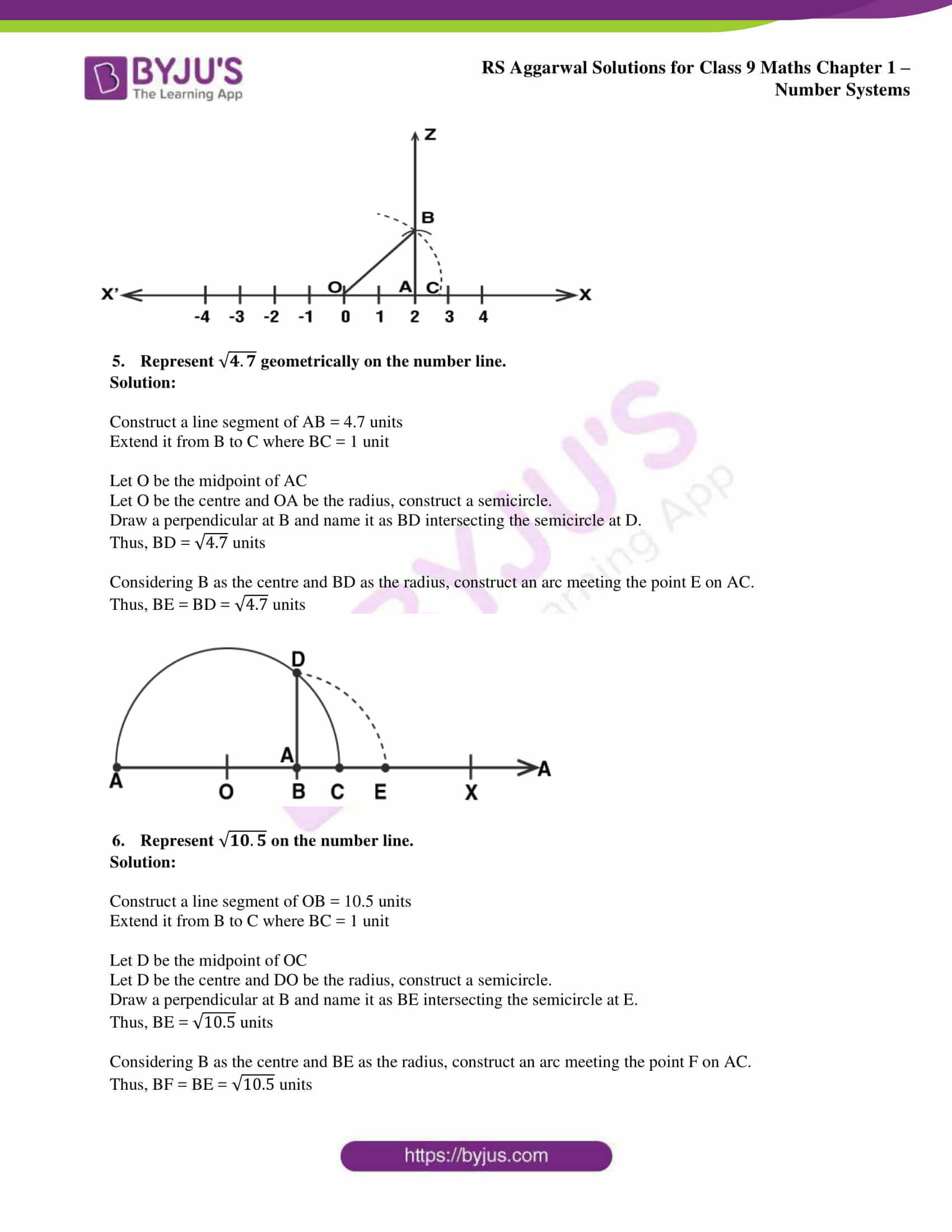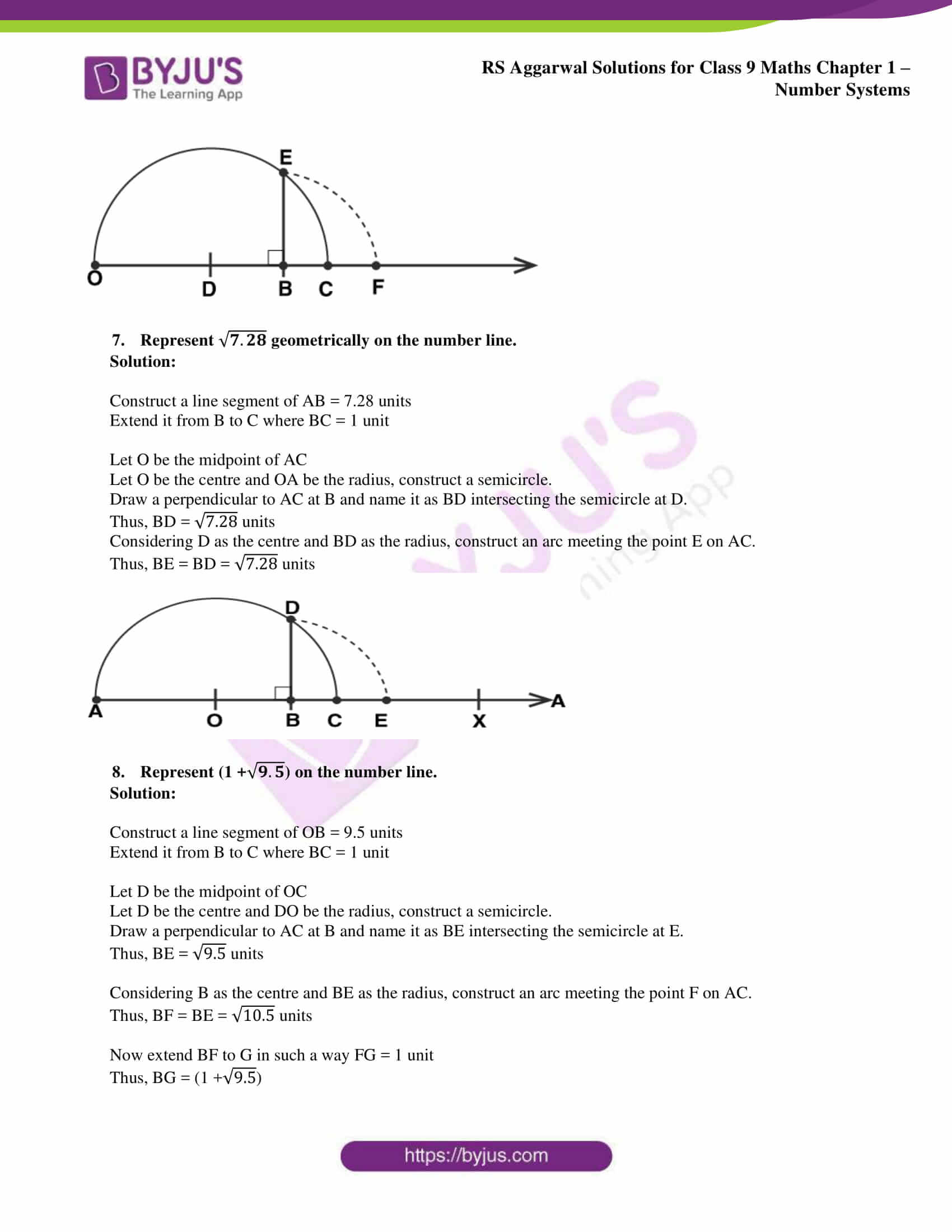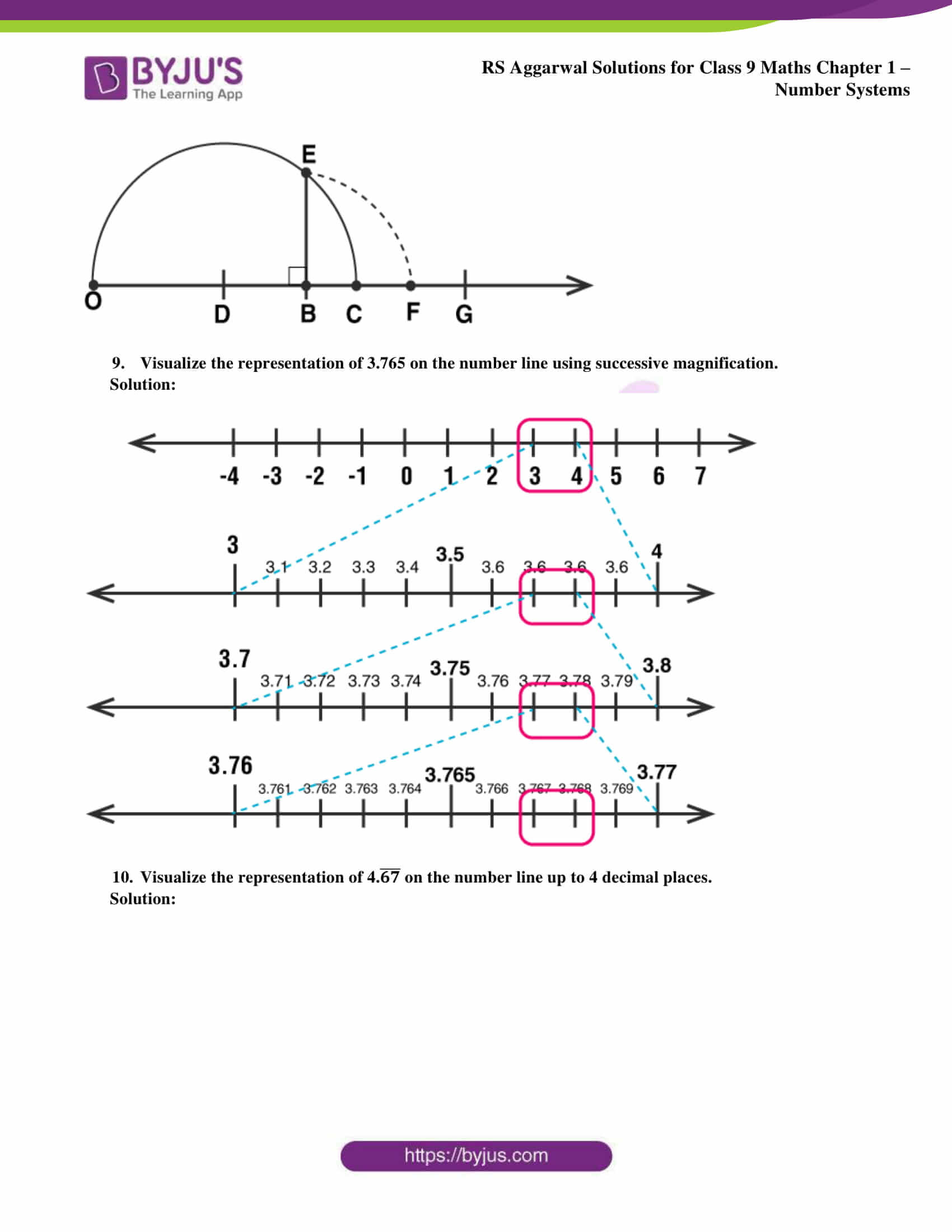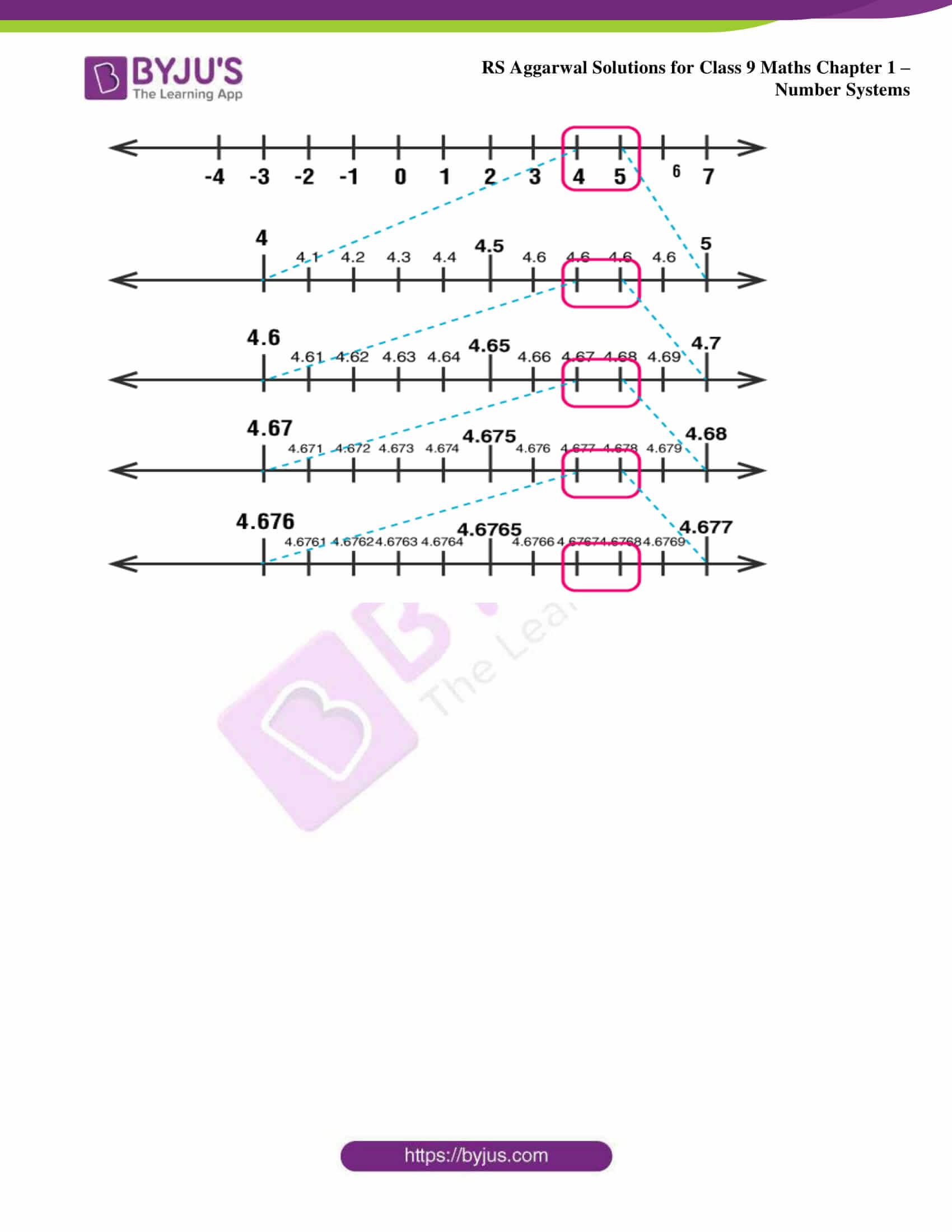### Access other exercise solutions of class 9 Maths Chapter 1: Number Systems

Exercise 1A Solutions 9 Questions

Exercise 1B Solutions 5 Questions

Exercise 1C Solutions 14 Questions

Exercise 1D Solutions 8 Questions

Exercise 1F Solutions 25 Questions

Exercise 1G Solutions 18 Questions

### RS Aggarwal Solutions Class 9 Maths Chapter 1 – Number Systems Exercise 1E

RS Aggarwal Solutions Class 9 Maths Chapter 1 Number Systems Exercise 1E tells us in brief about the methods used for the representation of real numbers and irrational numbers on a number line.

### Key features of RS Aggarwal Solutions for Class 9 Maths Chapter 1: Number Systems Exercise 1E

• The solutions prepared are for both CBSE syllabus and for state boards which are following the CBSE syllabus.
• Our team of experts having vast knowledge in Mathematics prepare solutions in an explanatory manner depending upon the board exams.
• Numerical and theoretical aspects of Mathematics are covered with exercise wise answers.
• Solved examples in RS Aggarwal Solutions for Class 9 help students in solving the exercise problems with ease.